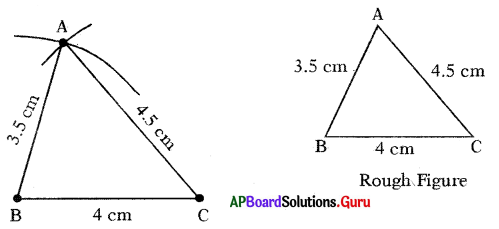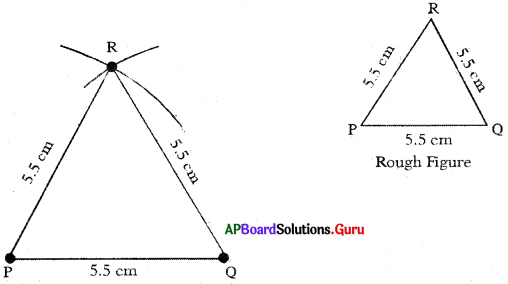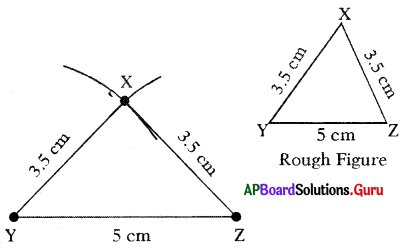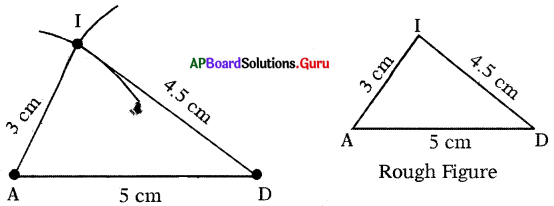SCERT AP 7th Class Maths Solutions Pdf Chapter 10 Construction of Triangles Ex 10.1 Textbook Exercise Questions and Answers.

## AP State Syllabus 7th Class Maths Solutions 10th Lesson Construction of Triangles Ex 10.1

Question 1.
Construct ∆ABC with measurements such that AB = 3.5 cm, BC = 4 cm and AC = 4.5 cm.
Given sides of ∆ABC are AB = 3.5 cm, BC = 4 cm and AC = 4.5 cm.Steps of Construction:

1. Draw a rough sketch of the triangle and label it with the given measurements.
2. Draw a line segment BC of length 4 cm.
3. Draw an arc with centre B and radius 3.5 cm.
4. Draw another arc with centre C and radius 4.5 cm to intersect the previous arc at A.
5. Join AB and AC.
Thus, required ∆ABC is constructed with the given measurements.Question 2.
Construct an equilateral triangle PQR with side PQ = 5.5 cm
Given sides of an equilateral triangle ∆PQR is PQ = 5.5 cm.
In an equilateral triangle all sides are equal in length.
∴ PQ = QR = PR = 5.5 cmSteps of Construction:

1. Draw a rough sketch of the triangle and label it with the given measurements.
2. Draw a line segment PQ of length 5.5 cm.
3. Draw an arc with centre P arid radius 5.5 cm.
4. Draw another arc with centre Q and the same radius (5.5 cm) to intersect the previous arc at R.
5. Join PR and QR.
Thus, required ∆PQR is constructed with the given measurements.

Question 3.
Construct ∆XYZ with measurements XY = 3.5 cm, YZ = 5 cm and ZX = 3.5 cm. Which type of triangle is this ? . ‘
Given sides of the ∆XYZ are XY = 3.5 cm, YZ 5 cm and ZX = 3.5 cm. In ∆XYZ two sides (XY = ZX = 3.5 cm) are equal.
So, ∆XYZ is an isosceles triangle.Steps of Construction:

1. Draw a rough sketch of the triangle and label it with the given measurements.
2. Draw a line segment YZ of length 5 cm.
3. Draw an arc with centre Y and radius 3.5 cm.
4. Draw another arc with centre Z and same radius (3.5 cm) to intersect the previous arc at X.
5. Join XY and XZ.
Thus, required ∆XYZ is constructed with the given measurements.Question 4.
Construct a triangle with measurements of 5 cm, 3 cm and 4.5 cm.